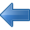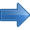Electrostatic Applications Books by Topic Author IndexPrevious Computer and mathematical models Next# Field Solutions on Computers

## Stanley J. Humphries, Jr., University of New Mexico

1998

400 pages

Field Solutions on Computers covers a broad range of practical applications involving electric and magnetic fields. The text emphasizes finite-element techniques to solve real-world problems in research and industry. After introducing numerical methods with a thorough treatment of electrostatics, the book moves in a structured sequence to advanced topics. These include magnetostatics with non-linear materials, permanent magnet devices, RF heating, eddy current analysis, electromagnetic pulses, microwave structures, and wave scattering. The mathematical derivations are supplemented with chapter exercises and comprehensive reviews of the underlying physics. The book also covers essential supporting techniques such as mesh generation, interpolation, sparse matrix inversions, and advanced plotting routines.

Contents
Introduction
Overview
Some Precautions
Finite-Element Electrostatic Equations
Coulomb's Law
Gauss's Law and Charge Density
Differential Equations for Electrostatic Fields
Charge Density Distributions and Dielectric Materials
Finite Elements
Coordinate Relationships for Triangles
Gauss's Law for Elements at a Vertex Point
Solution Procedure and Boundary Conditions
Electrostatics in Cylindrical Coordinates
Exercises
Minimum-Energy Principles in Electrostatics
Electrostatic Field Energy
Elements of the Calculus of Variations
Poisson Equation As a Condition of Minimum Energy
Finite-Element Equations for Two-Dimensional Electrostatics
Three-Dimensional Finite-Element Electrostatics on Arbitrary Meshes
Higher-Order Finite-Element Formulations
Exercises
Finite-Difference Solutions and Regular Meshes
Difference Operators
Initial Value Solutions of Ordinary Differential Equations
One-Dimensional Poisson Equation
Solution of the Poisson Equation by Back-Substitution
Two-Dimensional Electrostatic Solutions on a Regular Mesh
Three-Dimensional Electrostatic Solutions on a Regular Mesh
Exercises
Techniques for Numerical Field Solutions
Regular Meshes in Three Dimensions
Two-Dimensional Conformal Triangular Meshes
Fitting Triangular Elements to Physical Boundaries
Neumann Boundaries in Resistive Media
Boundary Value solutions by Successive Over-Relaxation
Exercises
Matrix Methods for Field Solutions
Introduction
Gauss-Jordan Elimination
Solving Tridiagonal Matrices
Matrix Solutions for One-Dimensional Electrostatics
Matrices for Two-Dimensional Finite-Element Solutions
Solving Tridiagonal Block Matrix Problems
Exercises
Analyzing Numerical Solutions
Locating Elements
Generalized Least-Square Fits
Field Calculations on a Two-Dimensional Triangular Mesh
Mesh and Boundary Plots
Contour, Element, Elevation, and Field Line Plots
Exercises
Nonlinear and Anisotropic Materials
Iterative Solutions to Boundary Value Problems
Numerical Data for Material Properties
Finite-Element Equations for Anisotropic Materials
Exercises
Finite-Element Magnetostatic Solutions
Differential and Integral Magnetostatic Equations
Vector Potential and Field Equations in Two Dimensions
Isotropic Magnetic Materials
Finite-Element Magnetostatic Equations
Magnetic Field Solutions
Properties of Permanent Magnet Materials
Magnetostatic Solutions with Permanent Magnets
Exercises
Static Field Analysis and Applications
Volume and Surface Integrals on a Finite-Element Mesh
Electric and Magnetic Field Energy
Capacitance Calculations
Inductance Calculations
Electric and Magnetic Forces on Materials
Charged-Particle Orbits
Electron and Ion Guns
Generalized Neumann Boundaries - Hall Effect Devices
Exercises
Low-Frequency Electric and Magnetic Fields
Maxwell Equations
Complex Numbers for Harmonic Quantities
Electric Field Equations in Resistive Media
Electric Field Solutions with Complex Number Potentials
Magnetic Fields with Eddy Currents
Exercises
Thermal Transport and Magnetic Field Diffusion
Thermal Transport Equation
Finite-Difference Solution of the Diffusion Equation
Finite-Element Diffusion Solutions
Instabilities in Finite-Element Diffusion Solutions
Magnetic Field Diffusion
Exercises
Electromagnetic Fields in One Dimension
Planar Electromagnetic Waves
Time-Domain Electromagnetism in One Dimension
Electromagnetic Pulse Solutions
Frequency-Domain Equations
Scattering Solutions
One-Dimensional Resonant Modes
Exercises
Two- and Three-Dimensional Electromagnetic Simulations
Introduction
Time-Domain Equations on a Conformal Mesh
Electromagnetic Pulse Solutions
Frequency-Domain Equations
Methods for Scattering Solutions
Waveguides and Resonant Cavities
Power Losses and Q Factors
Finite-Difference Time-Domain Method in Three Dimensions
Three-Dimensional Element-Based Time-Domain Equations
Exercises
Bibliography
Index